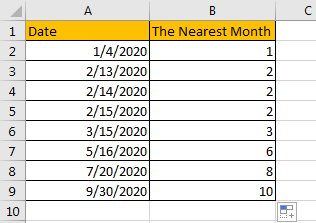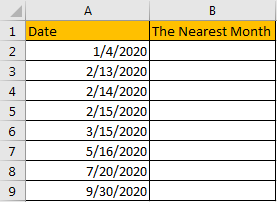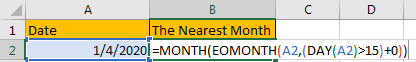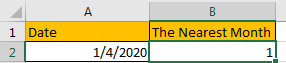# How to Round Off Date to the Nearest Month in Excel

Sometimes we may get some dates in excel and we want to calculate their nearest month based on date. Actually, this can be implemented by applying formula in excel. This tutorial will introduce you the formula to round off date to its nearest month.

Precondition:

Create a list contains some dates.## Method: Round Off Date to the Nearest Month by Formula

Step 1: In B2 enter the formula =MONTH(EOMONTH(A2,(DAY(A2)>15)+0)).

In this formula, EOMONTH function returns the date of the last day of a month before or after the specified date; MONTH function returns an integer between 1 and 12 which represents the month in a year. Use the combination of above two functions can round off date to its nearest month.Step 2: Press Enter to get returned value. Verify that for date 1/4/2020, the nearest month is January.Step 3: Drag down the fill handle till reaching the end of the list. Verify that all the nearest months are calculated properly.Comment:

1. This formula only returns an approximate value, no matter how many days a month, the nearest month for date >15 is the next month.
2. For special month like February, you can adjust the formula to match your request, for example if you want to get 3 (March) for date>13, you can update your formula =MONTH(EOMONTH(A4,(DAY(A4)>13)+0)).

### Related Functions

• Excel MONTH function
The Excel MONTH function returns the month of a date represented by a serial number. And the month is an integer number from 1 to 12. The syntax of the MONTH function is as below:=MONTH (serial_number)…
• Excel DAY function
The Excel DAY function returns a day of a date (from 1 to 31).The DAY function is a build-in function in Microsoft Excel and it is categorized as a DATE and TIME Function.The syntax of the DAY function is as below:= DAY (date_value)…